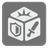Battle Dice
Moderate
English DE ES RU

For this task, you need to figure out what your probability of winning a board game is. The game involves two players moving units around a map. When the units battle and both players roll several dice, one for each unit, to see who wins and at what cost. You want to find the probability of winning one of these battles, regardless of any losses. Keep in mind that if the battle ends with no units remaining on either side, that's a draw, not a win.

When a conflict begins, each player rolls one die per unit. Each die has a number of attack icons and defense icons on each side. After a roll, the player loses a number of units equal to the number of attack icons the opponent rolled minus the number of defense icons they rolled themselves. For example, if player one rolled 2 attack icons and 4 defense icons and player two rolled 3 attack icons and 1 defense icons, player one would lose 0 units (3 - 4 but you can't lose negative units) and player two would lose 1 unit (2 - 1).

After unit losses are applied, the players roll again. This continues until one player has no units remaining.

You are given a description of the dice as a list of which icons are on a face and how many dice each player has. All of the dice are exactly the same. Each element in the list is a string containing zero or more A's representing the attack icons and zero or more D's representing the defense icons. (A face can be blank.) For example, the list ["AAD", "ADD", "A", "D", "", ""] represents a six sided die with two attack and one defense on one face, one attack and two defense on another, a single attack on the third face, a single defense on the fourth and two blank faces.

You should calculate the probability that player one will win the conflict. If player one has a 1 in 7 chance of winning, you should return ≈0.1429. The result should be given with four digits precision as ±0.0001.

Input: Three arguments. A dice description as a list of strings. A number of units for player one and player two as integers.

Output: The probability that player one will win the conflict as a float or integer.

Example:

```battle_probability(['A', 'D'], 3, 3) == 0.0000 # It's not immediately obvious, but each player will always lose the same number of units
battle_probability(['A', 'D'], 4, 3) == 1.0000
battle_probability(['AA', 'A', 'D', 'DD'], 3, 4) == 0.0186
battle_probability(['AA', 'A', 'D', 'DD'], 4, 4) == 0.4079
battle_probability(['AA', 'A', 'D', 'DD'], 5, 4) == 0.9073
```

How it is used: Such multi-step probability calculations are a staple of both programming contests and probability textbooks.

Preconditions:
1 ≤ units_one ≤ 10
1 ≤ units_two ≤ 10
2 ≤ len(dice_description) ≤ 10
There is at least 1 attack icon on the die
There are at most 3 icons on each face